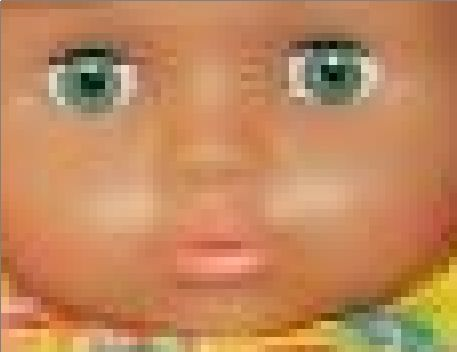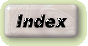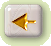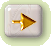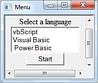Image-Downsize
by means of exact Pixel-Calculus in
Visual Basic
Nov 2007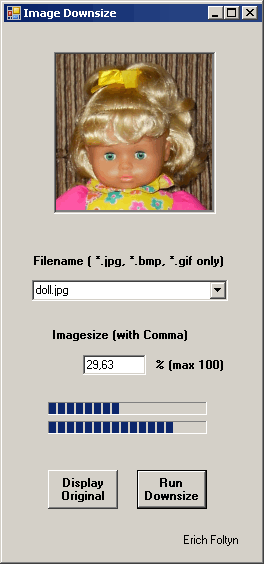Images, taken-up by a digital camera, are often needed to downsize for use in internet etc. By the downsize-procedure in available graphic-grograms often loss of picture-quality occurs. The following contribution tries to show the maximal attainable picture-quality by exact calculus of the pixels (pixel-colors) of the daughter-picture with uninteger pixel-sizes of the mother-picture. But here the intention is only to show the principle. In the example the processing by the exact calculation of the pixel-colors, is done by use of Visual Basic (VB). But this is paid by a long run-time for calculation, although VB is a compiler language. The calculation-time depends on the picture-size and can exceed over one minute for the picture-size of a digital-camera. Therefor I have used 2 progress bars on my form. It would have to be tested to calculate it by assembler-code in order to approach the realization, how the graphic-program-designers would make it. The goal is achieved in 4 steps: Step: The image is loaded form disk into a bitmap-image-object Step: The pixel-colors are picked out of the image-object and stored into an array Step: The array-values are processed in a downsize-procedure and the results stored in a second array Step: The colors from the second array are written back into the image-object, displayed and stored on disk

## Step 1

```          Try
image1 = New Bitmap(Fulname, True) : PictureBox1.Image = image1
Catch ex As ArgumentException
vbCrLf + "Check the path.", "Error")
End Try
```

## Step 2

```          Dim h As Integer = image1.Height : Dim w As Integer = image1.Width
For y = 0 To h - 1
For x = 0 To w - 1
Dim pixelColor As Color = image1.GetPixel(x, y)
Dim newColor1 As Color = Color.FromArgb(pixelColor.R, pixelColor.G, pixelColor.B)
ff = newColor1.ToArgb ' Format = aarrggbb in Decimal
For farbe = 3 To 1 Step -1
FarbeIn(x, y, farbe) = ff - 256 * Int(ff / 256) : ff = Int(ff / 256)
Next
Next
Next
```

## Step 3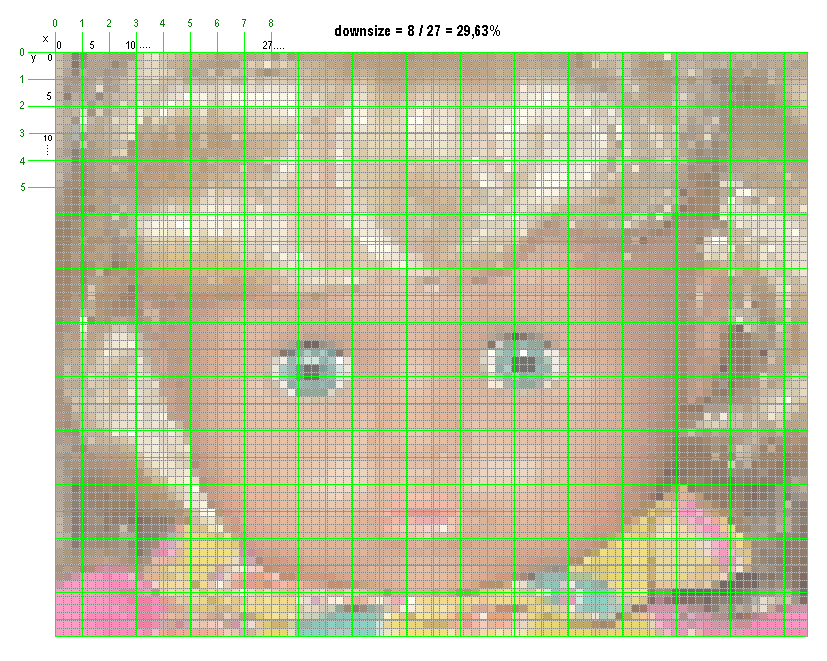in the squares of the green raster the calculation, shown in the following Visual-Basic-Code, delivers a medium pixel-color
```     ' h = heiht, w = width, v = downsizefactor in %
' Xd, Yd ... pixel-coordinates of the daughter-picture
' x1, y1 ... upper left corner of a green square
' x2, y2 ... lower right corner of a green square
' r1x, r2x, r1y, r2y .... xy coordinates within a pixel (of the mother-img)
' f = 1 (for rd), f = 2 (for gn), f = 3 (for bl)

d = 100 / v : Yd = -1

For y1 = 0 To h - 1 Step d : Yd += 1   ' Yd = Yd + 1
y2 = y1 + d : If y2 > h Then y2 = h
y10 = Int(y1) : y20 = Int(y2)
r1y = y1 - y10 : r2y = y2 - y20
Xd = -1
For x1 = 0 To w - 1 Step d : Xd += 1   ' Xd = Xd + 1
x2 = x1 + d : If x2 > w Then x2 = w
x10 = Int(x1) : x20 = Int(x2)
r1x = x1 - x10 : r2x = x2 - x20

' Square
For y = y10 To y20 : ky = 1
If y = y10 Then ky = 1 - r1y
If y = y20 Then ky = r2y
If ky < 0 Then ky = 0

For x = x10 To x20 : kx = 1
If x = x10 Then kx = 1 - r1x
If x = x20 Then kx = r2x
If kx < 0 Then kx = 0
For f = 1 To 3
Color(f) = Color(f) + kx * ky * ColorIn(x, y, f)
Next
Next
Next : xx = x2 - x1 : yy = y2 - y1
For f = 1 To 3
ColorOut(Xd, Yd, f) = CInt(Color(f) / (xx * yy))
Color(f) = 0
If ColorOut(Xd, Yd, f) < 0 Then ColorOut(Xd, Yd, f) = 0
If ColorOut(Xd, Yd, f) > 255 Then ColorOut(Xd, Yd, f) = 255
Next
'End of the square
Next
Next
```

## Step 4

```     For y = 0 To Yd
For x = 0 To Xd
rt = BildArrayOut(x, y, 1)
gn = BildArrayOut(x, y, 2)
bl = BildArrayOut(x, y, 3)
Dim newColor2 As Color = Color.FromArgb(rt, gn, bl)
image1.SetPixel(x, y, newColor2)
Next
Next
' Set the PictureBox on the form smaller if to display smaller pictures.
Dim y, x As Integer
x = width : y = height
If x > 660 Then x = 660
If y > 495 Then y = 495
PictureBox1.ClientSize = New Size(x, y)
PictureBox1.Image = CType(image1, Image)

image1.Save(NewFilename) ' Save Downsized File on Disk
```

In the following shown the result: Picture downsized by 2/7 = 28,57% as assumed in the example above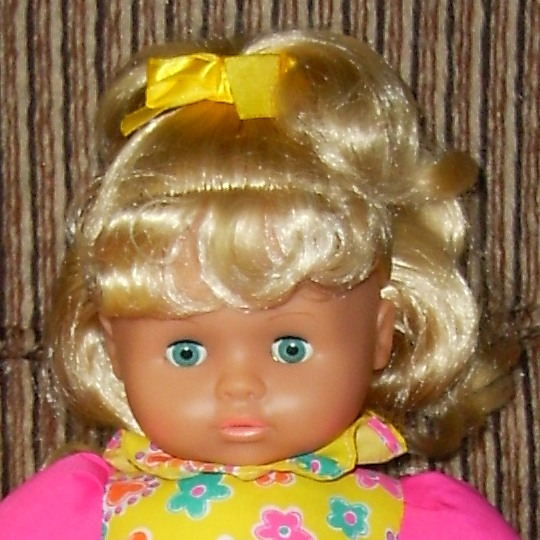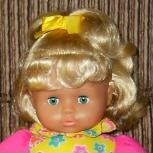In the following shown the details of the small picture, enlarged in the comparison with the large, rastered picture above: Visibly there is a loss of sharpness, certainly, but with exactly calculated pixel-colors. So the details in the small picture remain well visible and it seems as if the eyebrows are still there.